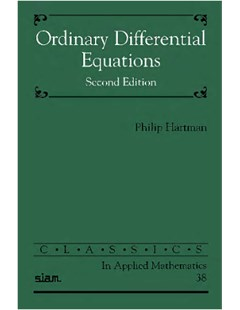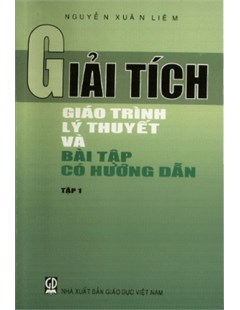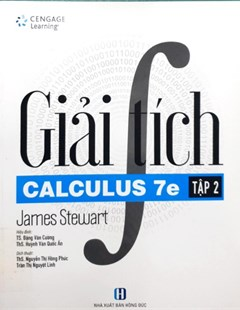Ordinary differential equations

Ordinary Differential Equations is based on the author's lecture notes from courses on ODEs taught to advanced undergraduate and graduate students in mathematics, physics, and engineering. The book, which remains as useful today as when it was first published, includes an excellent selection of exercises varying in difficulty from routine examples to more challenging problems. These exercises show extensions of the techniques in question and serve to introduce the reader to the literature in this area. New to this SIAM Classics edition is an Errata section listing corrections to minor errors in the 1982 edition. An extensive bibliography (up to 1980) is provided.

# Ordinary differential equations

2002

## Abstract

Ordinary Differential Equations covers the fundamentals of the theory of ordinary differential equations (ODEs), including an extensive discussion of the integration of differential inequalities, on which this theory relies heavily. In addition to these results, the text illustrates techniques involving simple topological arguments, fixed point theorems, and basic facts of functional analysis. Unlike many texts, which supply only the standard simplified theorems, Ordinary Differential Equations presents the basic theory of ODEs in a general way, making it a valuable reference.

This SIAM reissue of the 1982 second edition covers invariant manifolds, perturbations, and dichotomies, making the text relevant to current studies of geometrical theory of differential equations and dynamical systems. In particular, Ordinary Differential Equations includes the proof of the Hartman-Grobman theorem on the equivalence of a nonlinear to a linear flow in the neighborhood of a hyperbolic stationary point, as well as theorems on smooth equivalences, the smoothness of invariant manifolds, and the reduction of problems on ODEs to those on “maps” (Poincaré).

Contents:

• Preliminaries
• Existence
• Differential inequalities and uiniqueness
• Linear differential equations
• Dependence on initial conditions and parameters
• Total and partial differential equations
• The poincare-bendixon theory
• Plane stationary points
• Invariant manifolds and linearizations
• Pertubed linear systems
• Liner second order equations
• Use of implicit function and fixed point therems
• Dichotomies for solutions of linear equations
• Miscellany on monotony

## Citation

Philip Hartman, Ordinary differential equations, Society for industrial and applied mathematics philadelphia, 2002

Đại cương

## Related documentOrdinary differential equations Giải tích: Giáo trình lý thuyết và Bài tập có hướng dẫn giải – Tập 1 Giải tích Calculus 7e Tập 2

## QR code## Content

• Thứ Sáu, 09:59 06/05/2022

### Tin tiêu điểm

Tài nguyên giáo dục mở Việt Nam (Vietnam Open Educational Resources – VOER)

Thứ Sáu, 19:07 02/12/2022

Tài nguyên truy cập mở Digital Commons Network

Thứ Sáu, 15:15 04/11/2022

Giới thiệu bộ sưu tập lĩnh vực cơ khí, chế tạo máy

Thứ Tư, 10:29 19/10/2022

### Các bài đã đăng

Reflect 2: Listening & Speaking (Student's Book)

Thứ Sáu, 09:55 22/09/2023

Reflect 1: Reading & Writing (Teacher's guide)

Thứ Sáu, 09:43 22/09/2023

Thứ Sáu, 09:25 22/09/2023

Reflect 1: Listening & Speaking (Teacher's guide)

Thứ Sáu, 09:10 22/09/2023

Tìm hiểu văn hóa tâm linh của người Việt

Thứ Sáu, 08:33 22/09/2023

An Introduction To The Use Of The Keil C51 Compiler On The 8051 Family

Thứ Sáu, 09:36 06/05/2022

How to do Discourse Analysis: A Toolkit

Thứ Sáu, 09:27 06/05/2022

An Introduction to Discourse Analysis: Theory and Method (4th edition)

Thứ Sáu, 09:07 06/05/2022

Programming Language Pragmatics (Fourth Edition)

Thứ Sáu, 09:02 06/05/2022

Giải tích: Giáo trình lý thuyết và Bài tập có hướng dẫn giải – Tập 1

Thứ Năm, 18:06 05/05/2022